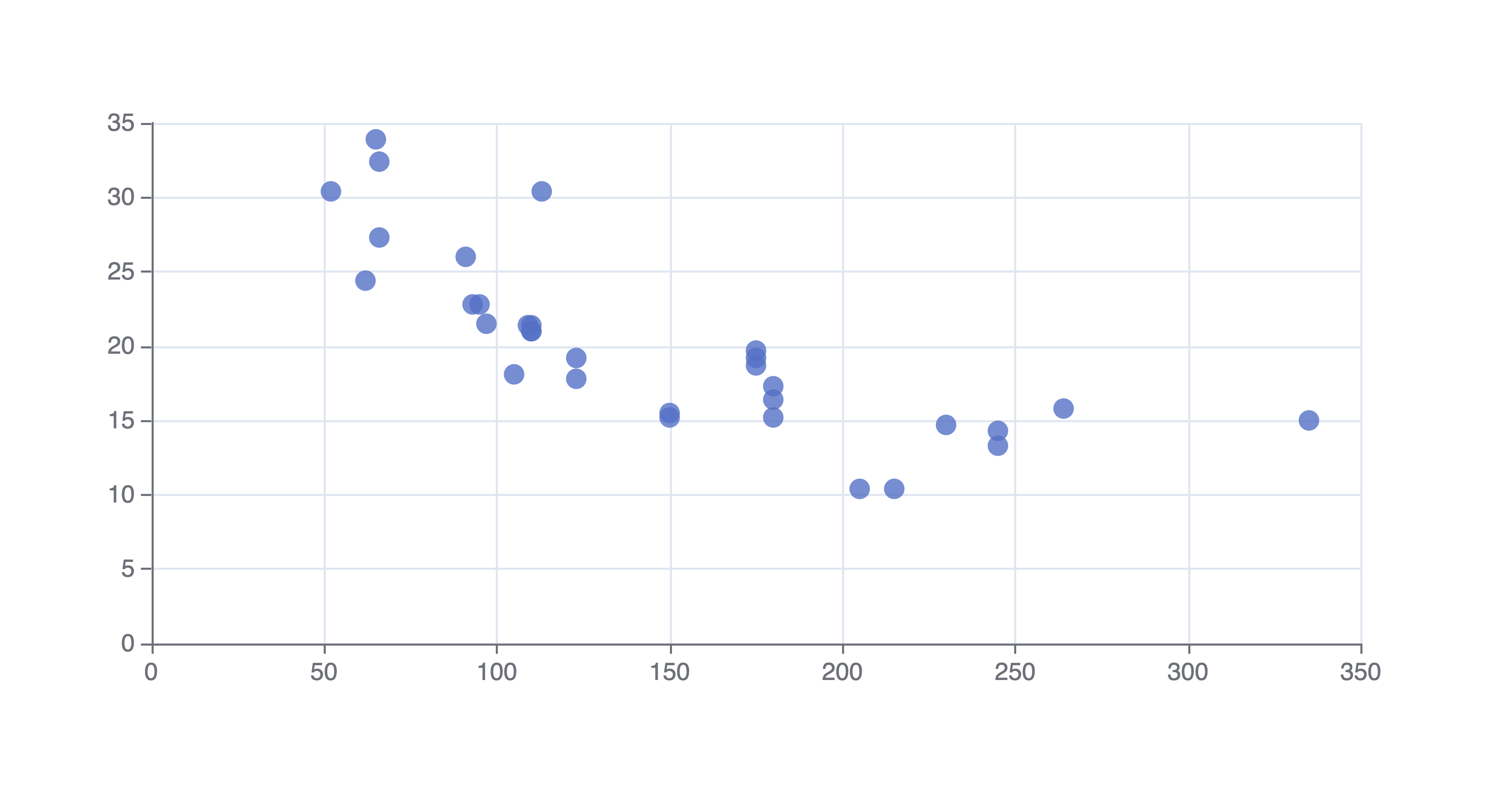# Scatter Plot

Or input your data as csv

Sharing helps us build more free tools

Quickly create a scatter plot with this free online scatter plot maker.

Scatter plots are useful for showing a correlation between two variables. They use continuous values on the y axis and continuous or discrete values on the x axis.A scatter plot is sometimes called a scatter chart, scatter graph, or scatter diagram.

## When To Use aScatter Plot

Scatter plots are useful for showing correlation when your data set is messy or large. A line graph can be a better option if you have a small amount of data with an obvious correlation. Line graphs can become very messy and hard to read when the data set is large.

Regression or correlation can be used to quantify the relationship in your data. You can even put your line of best fit on top of your scatter plot to show how it fits the data.

## How To Make a Scatter Plot

Click the dataset input at the top of the page. Or drag and drop your dataset into the input box.

2. ### Pick a column for the horizontal x-axis

Choose a column for the x values that are not affected by the column that you will use on the vertical y-axis. The scatter plot maker will create a scatter plot.

3. ### Pick a column for the vertical y-axis

Choose a column for the y values that are influenced by the x values. This will make it easier to see how the x values affect the y values.